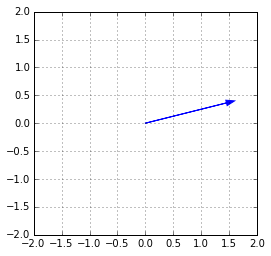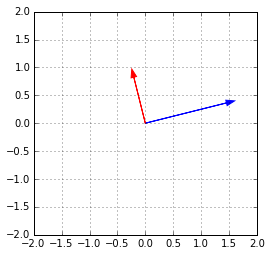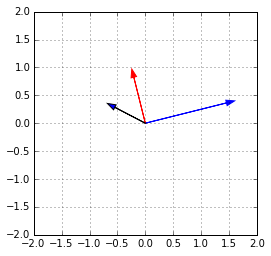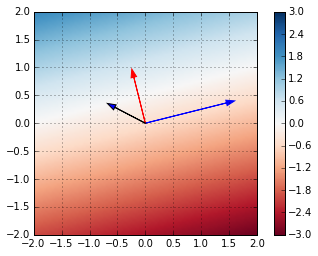# Point-normal form and signed distance

In :
import numpy as np
import numpy.linalg as la
import matplotlib.pyplot as pt

In :
def draw_arrow(v, **kwargs):

def set_up_plot():
pt.xlim([-2,2])
pt.ylim([-2,2])
pt.gca().set_aspect("equal")
pt.grid()


Let's grab some vector $v$:

In :
np.random.seed(18)
v = np.array([1.6, 0.4])
v

Out:
array([ 1.6,  0.4])

In :
set_up_plot()
draw_arrow(v, color="blue")Now let's find a unit normal vector $n$:

In :
b = np.random.randn(2)
b = b - b.dot(v)/v.dot(v)*v
n = b/la.norm(b, 2)


Check:

In :
print("Norm =", la.norm(n))
print("n.v =", n.dot(v))

Norm = 1.0
n.v = -2.77555756156e-16



Plot:

In :
set_up_plot()
draw_arrow(v, color="blue")
draw_arrow(n, color="red")Now let's grab a point $p$ and compute $r$:

In :
np.random.seed(355342)
p = np.random.randn(2)

r = p.dot(n)

In :
set_up_plot()
draw_arrow(v, color="blue")
draw_arrow(n, color="red")
draw_arrow(p)Next, evaluate signed distance on a grid of points in the plane:

In :
xs = np.linspace(-2, 2, 100)
ys = np.linspace(-2, 2, 100)

# make an grid of points in the plane
xys = np.empty((2, 100, 100))
xys = xs
xys = xs.reshape(-1, 1)

signed_dist = (
xys*n + xys*n - r
)

In :
set_up_plot()
draw_arrow(v, color="blue")
draw_arrow(n, color="red")
draw_arrow(p)

pt.imshow(signed_dist, extent=(-2, 2, 2, -2), cmap="RdBu", vmin=-3, vmax=3)
pt.colorbar()

Out:
<matplotlib.colorbar.Colorbar at 0x7fd9857736a0>• Where is the line parallel to $v$ through $p$? (that satisfies $x\cdot n -r = 0$)?

(Hint: Look for plot values = 0.)

• Can you read off the distance of the line from the origin?
• Can you read off the distance of the line from $v$?
• Can you read off the distance between the line and the top left corner of the plot?
• How was each such distance computed?
In []: